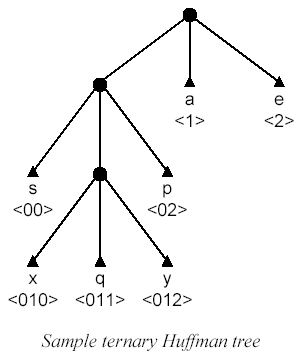Online JudgeProblem SetAuthorsOnline ContestsUser
Web Board
F.A.Qs
Statistical Charts
Problems
Submit Problem
Online Status
Prob.ID:
Register
Authors ranklist
Current Contest
Past Contests
Scheduled Contests
Award Contest
Register
Language:
Huffman Trees
 Time Limit: 1000MS Memory Limit: 10000K Total Submissions: 923 Accepted: 155

Description

A relatively simple method for compressing data works by creating a so-called Huffman tree for a file and using it to compress and decompress the data it contains. For most applications,binary Huffman trees are used (i.e., each node is either a leaf or has exactly two sub-nodes). One can, however, construct Huffman trees with an arbitrary number of sub-trees (i.e, ternary or, in general, N-ary trees).
A Huffman tree for a file containing Z different characters has Z leaves. The path from the root to a leaf that represents a certain character determines its encoding; each step towards the leaf determines an encoding character (which can be 0, 1, . . . , (N - 1)). By placing often-occurring characters closer to the root, and less often occurring characters further away from the root, the desirable compression is achieved. Strictly speaking, such a tree is a Huffman tree only if the resulting encoding takes the minimal number of N-ary symbols to encode the complete file.
For this problem, we only consider trees where each node is either an internal node, or a leaf encoding a character; there are no dangling leaves that do not encode for a character.The figure below shows a sample ternary Huffman tree; the characters 'a' and 'e' are encoded using one ternary symbol; the less frequently occurring characters 's' and 'p' are encoded using two ternary symbols; and the very rare symbols 'x', 'q' and 'y' are encoded using three ternary symbols each.Of course, if we want to decompress a list of N-ary symbols later on, it is important to know which tree was used to compress the data. This can be done in several ways. In this problem we use the following method: the stream of data will be preceded by a header consisting of the encoded versions of the Z characters occurring in the original file, in lexicographical order.

Given the number of source symbols Z, a value N denoting the 'arity' of the Huffman tree, and a header, give the mapping from source symbols to encoded symbols.

Input

The input starts with a single integer T on a separate line, denoting the number of test cases that follow. Next, for each of the T test cases, three lines follow:
 The number of different characters in the file (2 <= Z <= 20);
 The number N, denoting the 'arity' of the Huffman tree (2 <= N <= 10);
 A string representing the header of the received message; each character will be a digit in the range [0, (N -1)]. This string will contain less than 200 characters.
Note: Although rare, it is possible for a header to have multiple interpretations (e.g., the header '010011101100' with Z = 5 and N = 2). It is guaranteed that all cases in the test input have a unique solution.

Output

For each of the T test-cases, print Z lines giving the encoded version of each of the Z characters in the testcase in ascending order. Use the format original-> encoding, where original is a decimal value in the range [0, (Z -1)], and encoding is the string of encoding digits for this symbol (each digit is >= 0 and < N).

Sample Input

3
3
2
10011
4
2
000111010
19
10
01234678950515253545556575859

Sample Output

0->10
1->0
2->11
0->00
1->011
2->1
3->010
0->0
1->1
2->2
3->3
4->4
5->6
6->7
7->8
8->9
9->50
10->51
11->52
12->53
13->54
14->55
15->56
16->57
17->58
18->59

Source

[Submit]   [Go Back]   [Status]   [Discuss]Home PageGo BackTo top